Next: Sullivan's approach Up: Methods of calculation of Previous: Estimating forces from

# Corresponding semi-markovian chain

Since the process is markovian, one can write: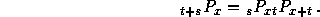involving elementary stochastic matriceswhich can be directly deduced from observed data. The LSOA survey uses basic matrices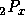instead of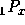, therefore each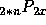matrix can be directly computed by simple products of matrix.

Most important outputs concern life expectancies which are expressed inas integer on time of a function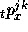giving the status at time t. But this function is known for each two year period, so life expectancies can be computed directly from the data without having to estimate forces.

Nicolas Brouard
Tue Jun 6 00:15:46 DF 1995